Question

# At the beginning of 2020 you invest \$3,000 in Microsoft (MSFT) stock and \$5,000 in Proctor...

At the beginning of 2020 you invest \$3,000 in Microsoft (MSFT) stock and \$5,000 in Proctor and Gamble (PG) stock.
Suppose you expect the monthly return to be 1.2% for MSTF and 0.5% for PG and the standard deviation of monthly returns to be 11.6% for MSTF and 6.5% for PG, and that the correlation of the returns on the two stock is 0.18.

- What are the expected monthly return on your portfolio and its stand dard deviation?

- Compare and discuss the return and standard deviation of the portfolio to the return and standard deviation of the two individual stocks.

Percentage Invested in Microsoft = (3000 / 8000) = 37.5%

Percentage Invested in Procter & gamble = (5000 / 8000) = 62.5%

Monthly return for Microsoft = 1.2%

Monthly return for Procter & gamble = 0.5%

Expected monthly return on portfolio = ( Weight of Stock A * Return of Stock A ) + ( Weight of Stock B * Return of Stock B )

= (0.375 * 1.2%) + (0.625 * 0.5%) = 0.76%

Expected standard deviation of portfolio = [ { ( Weight of Stock A * Std Deviation of Stock A) ^ 2 } + { ( Weight of Stock B * Std Deviation of Stock B) ^ 2 } + 2 * Weight of Stock A * Weight of Stock B * Correlation of Stock A & B * Std Deviation of Stock A * Std Deviation of Stock B ] ^ (1/2)

= 6.46%

Portfolio return will lie between Returns of 2 Stocks while Standard Deviation reduces to 6.46% on account of benefit of diversification

#### Earn Coins

Coins can be redeemed for fabulous gifts.

Similar Homework Help Questions
• ### 2. Go to Yahoo Finance, and search for Microsoft (MSFT). Click on historical data tab, and...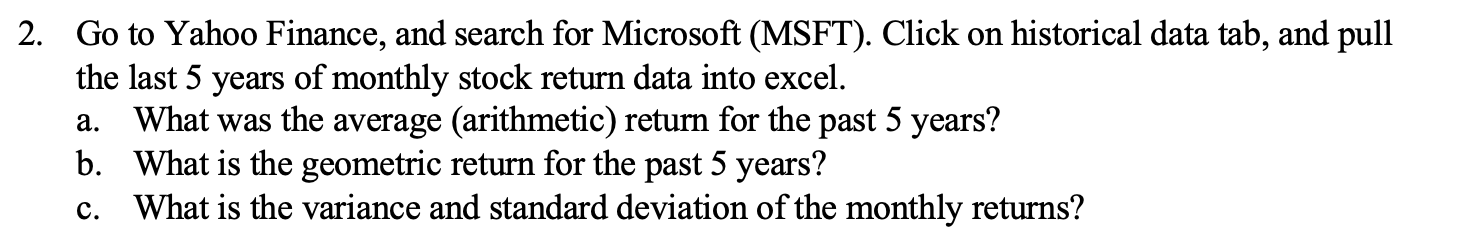2. Go to Yahoo Finance, and search for Microsoft (MSFT). Click on historical data tab, and pull the last 5 years of monthly stock return data into excel. a. What was the average (arithmetic) return for the past 5 years? b. What is the geometric return for the past 5 years? c. What is the variance and standard deviation of the monthly returns?

• ### Expected Returns 0.17 0.11 0.30 Standard Deviation 0.12 0.05 Firm A's common stock Firm B's common...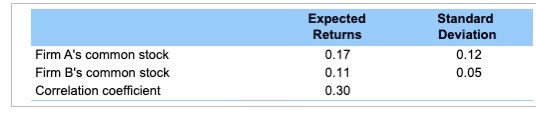Expected Returns 0.17 0.11 0.30 Standard Deviation 0.12 0.05 Firm A's common stock Firm B's common stock Correlation coefficient (Computing the standard deviation for a portfolio of two risky investments) Mary Guilott recently graduated from college and is evaluating an investment in two companies' common stock. She has collected the following information abou the common stock of Firm A and Firm B: a. If Mary decides to invest 10 percent of her money in Firm A's common stock and 90...

• ### You invest \$18,000 in a stock portfolio with an expected return of 14% and a standard...

You invest \$18,000 in a stock portfolio with an expected return of 14% and a standard deviation of 24%. You invest \$12,000 in a bond portfolio with an expected return of 6% and a standard deviation of 12%. The correlation between the two funds is 0.45. What is the standard deviation of the resulting portfolio?

• ### 6. Calculating a beta coefficient for a single stock Suppose that the standard deviation of returns...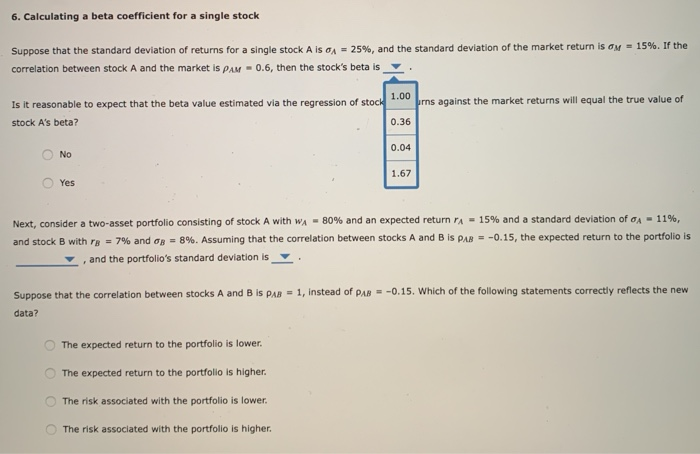6. Calculating a beta coefficient for a single stock Suppose that the standard deviation of returns for a single stock A IS A = 25%, and the standard deviation of the market return is on = 15%. If the correlation between stock A and the market is PAM - 0.6, then the stock's beta is prns against the market returns will equal the true value of Is it reasonable to expect that the beta value estimated via the regression of...

• ### Stocks A and B have the following returns Stock A 0.10 0.07 0.15 -0.05 0.08 Stock...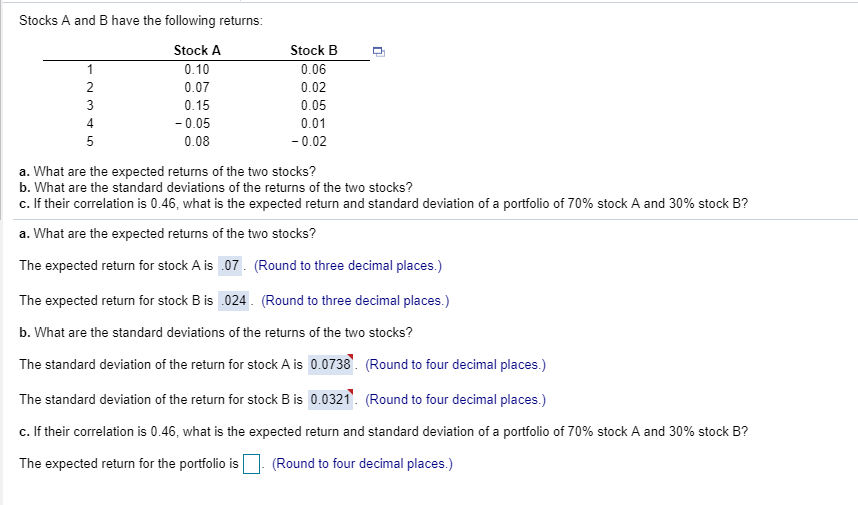Stocks A and B have the following returns Stock A 0.10 0.07 0.15 -0.05 0.08 Stock B 0.06 0.02 0.05 0.01 -0.02 4 a. What are the expected returns of the two stocks? b. What are the standard deviations of the returns of the two stocks? c. If their correlation is 0.46, what is the expected return and standard deviation of a portfolio of 70% stock A and 30% stock B? a. What are the expected returns of the two...

• ### P.14 An investor holding a portfolio consisting of two stocks invests 25% of assets in Stock...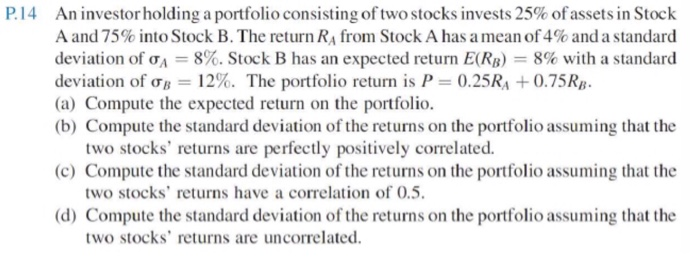P.14 An investor holding a portfolio consisting of two stocks invests 25% of assets in Stock A and 75% into Stock B. The return RA from Stock A has a mean of 4% and a standard deviation of A = 8%. Stock B has an expected return E(RB) = 8% with a standard deviation of ob = 12%. The portfolio return is P = 0.25RA +0.75RB. (a) Compute the expected return on the portfolio. (b) Compute the standard deviation of...

• ### please answer 6. Calculating a beta coefficient for a single stock Aa Aa Suppose that the...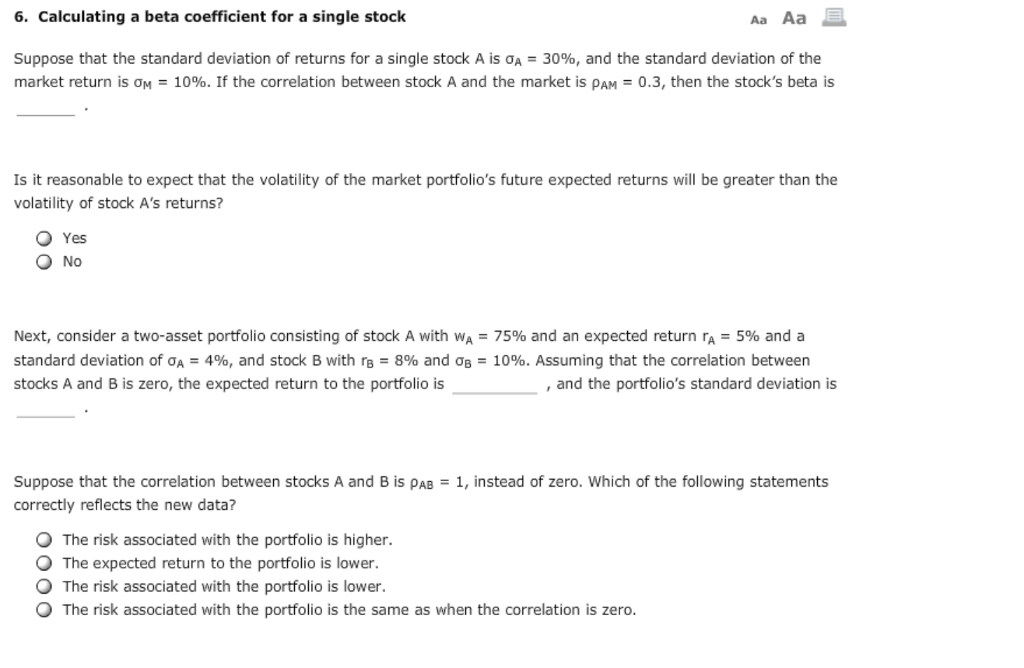please answer 6. Calculating a beta coefficient for a single stock Aa Aa Suppose that the standard deviation of returns for a single stock A is σΑ-30%, and the standard deviation of the market return is 얘-10%. If the correlation between stock A and the market is ρΑΜ-0.3, then the stock's beta is Is it reasonable to expect that the volatility of the market portfolio's future expected returns will be greater than the volatility of stock A's returns? O Yes...

• ### please answer 6. Calculating a beta coefficient for a single stock Aa Aa Suppose that the...please answer 6. Calculating a beta coefficient for a single stock Aa Aa Suppose that the standard deviation of returns for a single stock A is σΑ-30%, and the standard deviation of the market return is 얘-10%. If the correlation between stock A and the market is ρΑΜ-0.3, then the stock's beta is Is it reasonable to expect that the volatility of the market portfolio's future expected returns will be greater than the volatility of stock A's returns? O Yes...

• ### Suppose the expected returns and standards deviations of two stocks were stock A: E (R) =9%,...

Suppose the expected returns and standards deviations of two stocks were stock A: E (R) =9%, STANDARD DEVIATION = 36% STOCK B: E (R) = 15%, STANDARD DEVIATION = 62% A. calculate the expected return of a portfolio that is composed of 35% of stock A and 65% of stock B. b. calculate the standard deviation of this portfolio when the correlation coefficient between the returns is 0.5 c. calculate the standard deviation of this portfolio (same weights in each...

• ### Thank you... Using the data from the table what is the volatility of an equally weighted...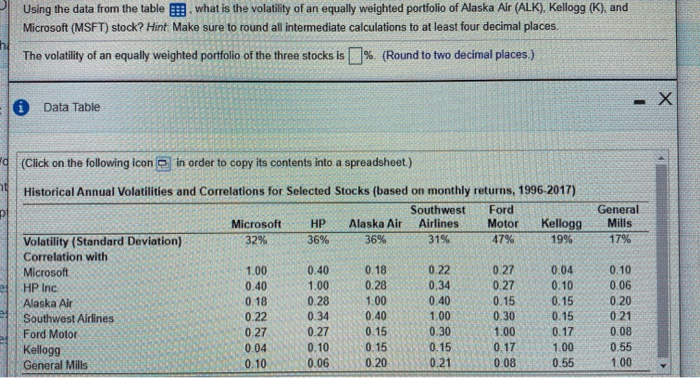Thank you... Using the data from the table what is the volatility of an equally weighted portfolio of Alaska Air (ALK), Kellogg (K), and Microsoft (MSFT) stock? Hint Make sure to round all intermediate calculations to at least four decimal places. The volatility of an equally weighted portfolio of the three stocks is %. (Round to two decimal places.) Data Table (Click on the following icon in order to copy its contents into a spreadsheet.) General Mills 179% Historical Annual...